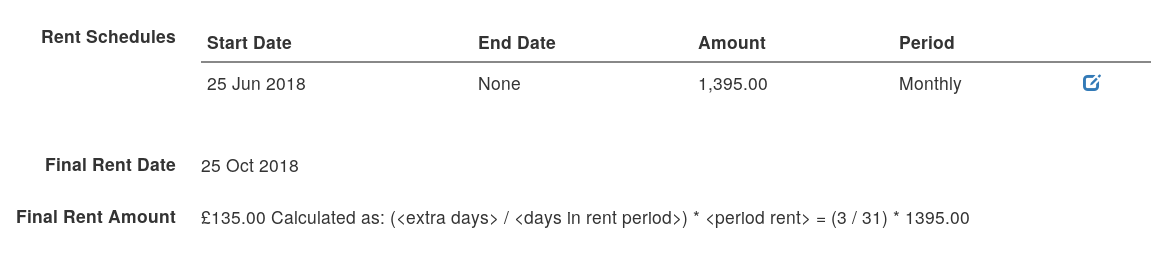# Feature Focus: Final Rent Calculation

When a tenancy comes to an end, it's always easiest if that aligns with a rent day - meaning that no partial month/week payment is due. However that's often not the case in the real world. Hence you'll find yourself calculating the rent due for the partial period used.

I've seen a few different ways of calculating this but the two most common are using a proportion of the current rental period and based on a day rate averaged over a year.

PaTMa uses the former of these, as it seems to be the most common and feels more "obvious" to most people.

## The Formula

``````([extra days] / [days in rent period]) * [period rent] = [final payment]
``````

This works out the proportion of days used in the rental period and multiplies it by the rent amount. Hence providing the proportion of the rent that's due.

Note: this is equivalent to working out the rent per day in the affected rental period and multiplying it by the number of days used. Ie ([period rent] / [days in rent period]) * [extra days]

PaTMa has always used this formula to calculate the final rental payment due, but it now also shows you the formula right next to the result. It also shows a version of the formula with all the numbers filled in so you can really understand where the final rent figure is coming from. Making it easy to access and also explain to your tenants if you need to.## The Other Way

Just in case you're curious, here's the formula for the other common calculation I've seen for final rent payments:

``````(([period rent] * [periods in year]) / 365) * [extra days] = [final payment]
``````

This approach only really works for monthly rental periods as there are exactly 12 in a year, whereas there aren't exactly 52 weeks in a year.

Scaling the rent up to a full year before then scaling it back down to a daily amount also seems more complicated than needed.

## The Simple Life

Would you rather not worry about how to calculate a final rent payment? You can manage a single unit for free with PaTMa and start simplifying your property management immediately.